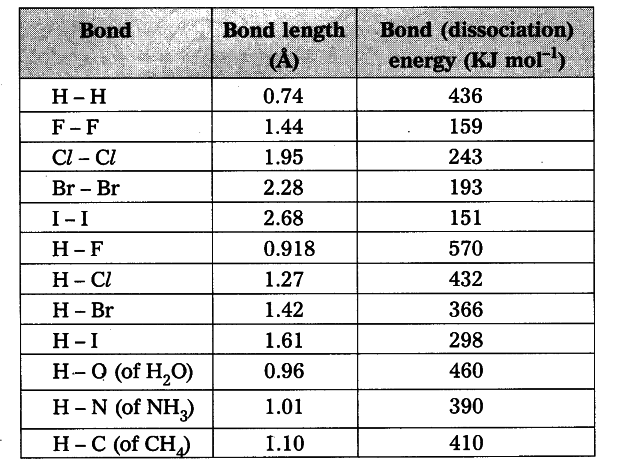# How bond energies and bond lengths of molecule helps us in predicting their chemical properties?

How bond energies and bond lengths of molecule helps us in predicting their chemical properties ? Explain with examples.

1. Bond length: Bond length or bond distance is the equilibrium distance between the nuclei of two atoms which form a covalent bond.
2. Bond energy : Bond energy or bond dissociation energy is the energy needed to break a covalent bond between two atoms of a diatomic covalent compound in its gaseous state.
3. If the nature of the bond between the same two atoms changes, the bond length also changes. For example, the bond lengths between two carbon atoms are :
C-C > C = C > C = C
4. Thus the various bond lengths between the two carbon atoms are in ethane 1.54 \overset { 0 }{ A }, ethylene 1.34 \overset { 0 }{ A }, acetylene 1.20 \overset { 0 }{ A }.
5. The bond lengths between two oxygen atoms are in \$H _{ 2 }\$\$O _{ 2 } (O - O) is 1.48 \overset { 0 }{ A }\$ and in O _{ 2 } (O = O) is 1.21 \overset { 0 }{ A }.
6. Observe the table.1. When bond length decreases, then bond dissociation energy increases.
2. When bond length increases, then bond dissociation energy decreases.
3. Bond length of H - H in H _{ 2 } molecule is 0.74 \overset { 0 }{ A } and its bond dissociation energy is 436 kJ/mol, whereas bond length of F - F in F _{ 2 } molecule is 1.44 \overset { 0 }{ A } and its bond dissociation energy is 159 kJ/mol.
4. Melting and boiling points of substances also can be determined by this bond energies and bond lengths.